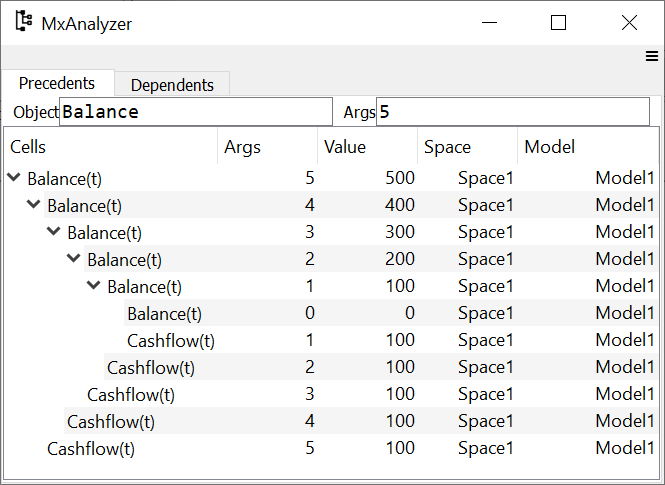# Features

## No programming

modelx enables you to build models just by defining Formulas like you do on spreadsheets. You can define Formulas by writing Python functions. modelx automatically resolves the calculation order from their dependency, so you don’t need to write scripts to run your models. modelx calculates the results when they are retrieved for the first time, and the results are kept until they are cleared or recalculated.

``````>>> import modelx as mx

>>> @mx.defcells
... def Cashflow(t):
...     return 100

>>> @mx.defcells
... def Balance(t):
...     if t > 0:
...         return Balance(t-1) + Cashflow(t)
...     else:
...         return 0

>>> Balance(5)
500

>>> dict(Balance)
{0: 0,
1: 100,
2: 200,
3: 300,
4: 400,
5: 500}
``````

Formulas are defined by Python functions, so you can enjoy the expressivenss of Python syntax. Python functions are far more readable, understandable than spreadsheet formulas.

Python have rich control flows, and built-in data structures such as list and dict. All of these are avaialble for defining Formulas.

Objects are referenced by their names in Formulas. You can also use lambda expressions to define simple Formulas.

## Object-oriented

modelx is object-oriented. You create, access or make changes to objects, such as Models, Spaces and Cells. modelx features composition and inheritance mechanisims common in Object-oriented programming (OOP).

``````
graph TD
A(Model1) --- B[Space1]
B --- C[Cells1]
B --- D[Space2]
D --- E(Cells2)
``````## Parameterization

Parameterization is a very powerful feature to quickly and naturally extend a Space written in terms of one combination of input values into a parameterized Space accepting arbitrary combinations of input values as arguments.

Using Space parameterization, you can parameterize Formula calculations without changing their signatures.

``````>>> space = mx.new_space("Loan")

>>> @mx.defcells
... def Payment():
...     return 1000 * Rate/100 * (1+Rate/100)**Term / ((1+Rate/100)**Term -1)

>>> space.parameters = ("Rate", "Term")

>>> space[3, 10].Payment()
117.23050660515952

>>> for rate, term in zip((3, 4, 5), (10, 20, 30)):
...     print(space[rate, term].Payment())
117.23050660515952
73.58175032862884
65.05143508027656
``````## Dependency tracing

Dependency tracing is an essential feature for checking and validating models. You can check what other values each calculated value is using, and also what other values it is used by.

``````>>> Balance.preds(5)
[Model1.Space1.Balance(t=4)=400, Model1.Space1.Cashflow(t=5)=100]

>>> Balance.succs(4)
[Model1.Space1.Balance(t=5)=500]
``````## Excel Interface

Excel files are great for storing data of relatively small sizes. You can create new spaces and pupulate new cells in the space with data from Excel files.

## GUI as Spyder plugin

Spyder is a popular open-source Python IDE. Spyder plugin for modelx adds custom IPython consoles and GUI widgets to use modelx with Spyder more intuitively.

## Version control

modelx models are saved as text files written in the Python syntax, which means you can take full advantage of modern version control systems and collaborative software development platforms, such as Git and GitHub.

## Document integration

Documenting models is an integral part of model governance. modelx enables you to document model components, such as Models, Spaces and Cells by setting their doc properties. When they are written to files, they are represented by Python modules and functions, and their doc propeties are represented by the docstrings of the Python modules and functions. Using Sphinx, a documentation generator widely used in the Python community for technical documentation, you can generate model documents from the docstrings, beautifully rendered in HTML, PDF and other formats.

See lifelib pages for document samples. Sphinx interpretes reStructuredText, a plaintext markup syntax, and can render math expressions written in LaTex, images, code samples as well as basic sets of markups, such as lists and links.